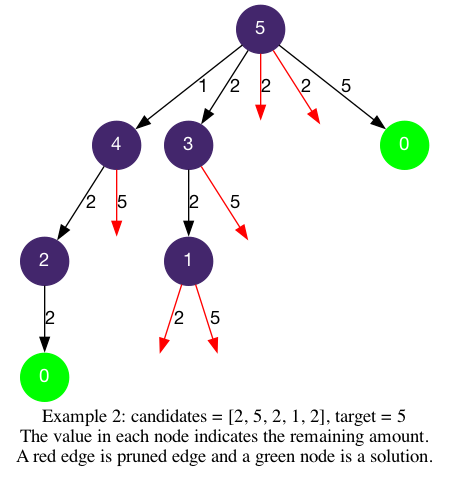# LeetCode Combination Sum II Solution

Given a collection of candidate numbers (`candidates`) and a target number (`target`), find all unique combinations in `candidates` where the candidate numbers sum to `target`.

Each number in `candidates` may only be used once in the combination.

Note: The solution set must not contain duplicate combinations.

Example 1:

Input: `candidates = [10,1,2,7,6,1,5], target = 8`

Output: `[ [1,1,6], [1,2,5], [1,7], [2,6] ]`

Example 2:

Input: `candidates = [2,5,2,1,2], target = 5`

Output: `[ [1,2,2],  ]`

Constraints:

• `1 <= candidates.length <= 100`
• `1 <= candidates[i] <= 50`
• `1 <= target <= 30`

## Solution

The logics for the template are:

• `start_index`: the start index of the next potential candidate in `candidates`.
• `is_leaf`: if `remaining == 0` we have reached our target using elements in `path`.
• `get_edges`: all candidates in `candidates[start_index:]`.
• `is_valid`: `candidate[i]` is valid if it does not exceed remaining and is not a duplicate.

We will follow the same steps as in Deduplication to validate and avoid a duplicate candidate.

#### Implementation

``````1def combinationSum2(self, candidates: List[int], target: int) -> List[List[int]]:
2    def dfs(start_index, path, remaining):
3        if remaining == 0:
4            ans.append(path[:])
5        for i in range(start_index, len(candidates)):
6            if remaining - candidates[i] < 0:   # avoid results exceeding target
7                break
8            elif i != start_index and candidates[i] == candidates[i-1]: # avoid duplicates
9                continue
10            path.append(candidates[i])
11            dfs(i+1, path, remaining - candidates[i])
12            path.pop()
13    candidates.sort()
14    ans = []
15    dfs(0, [], target)
16    return ans``````